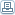# A New ATOMIC Model and Some Few Possible Applications

 By: Florian Ion Tiberiu PetrescuThe paper "A New ATOMIC Model And Some Few Possible Applications" presents, shortly, a new and original relation (18) to calculate the radius with that, the electron is running around the nucleus of an atom. One utilizes, two times the Lorentz relation (5), the Niels Bohr general equation (7), and a mass relation (4) which it was deduced from the kinematics energy relation written in two mode: classical (1) and coulombian (2). Equalizing the mass relation (4) with Lorentz relation (5) one obtains the equation (6), which give us a relation between the electron speed at power 2 (v2) and the radius (r). The second relation (8), between v2 and r, it was obtained by equalizing the mass of Bohr equation (7) and the mass of Lorentz relation (5). In the system (8) – (6) eliminating the electron speed (v2), one determines the radius r, with that, the electron is moving around the nucleus; see the relation (18). For a Bohr energetically level (n=a constant value), one determines now two energetically below levels, which form an electronic layer (a cloud). The author realizes by this paper a new atomic model. See the paper from one of these websites: